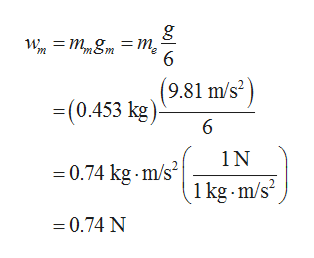# - A bag of sugar weighs 1.00 lb on Earth. What would it weigh in newtons on the Moon, where the free-fall acceleration is one-sixth that on Earth?  -Repeat for Pluto, where g is 0.665 times that on Earth. -Find the mass of the bag of sugar in kilograms at each of the three locations.Earth= ?kgMoon= ?kgPluto=?kg

Question
50 views

- A bag of sugar weighs 1.00 lb on Earth. What would it weigh in newtons on the Moon, where the free-fall acceleration is one-sixth that on Earth?

-Repeat for Pluto, where g is 0.665 times that on Earth.

-Find the mass of the bag of sugar in kilograms at each of the three locations.

Earth= ?kg

Moon= ?kg

Pluto=?kg

check_circle

Step 1

Consider the weight of the bag on Earth be we, the mass of the bag on Earth be me, the weight of the bag on Moon be wm, the mass of the bag on Moon be mm, the weight of the bag on Pluto be wp, and the mass of the bag on Pluto be mp.

The weight of the bag on the Earth is,

Step 2

The mass of the bag on the Earth can be calculated as,

Step 3

Since, the mass of an object does not vary, the mass of the bag is same at all th...help_outlineImage Transcriptioncloseи, — тЯт т, m (9.81 m/s -(0.453 kg) 6 1N 0.74 kg m/s2 1 kg m/s2 =0.74 N fullscreen

### Want to see the full answer?

See Solution

#### Want to see this answer and more?

Solutions are written by subject experts who are available 24/7. Questions are typically answered within 1 hour.*

See Solution
*Response times may vary by subject and question.
Tagged in

### Gravitation